AP and GP mix questions

Chapter 9 Class 11 Sequences and Series (Term 1)
Concept wise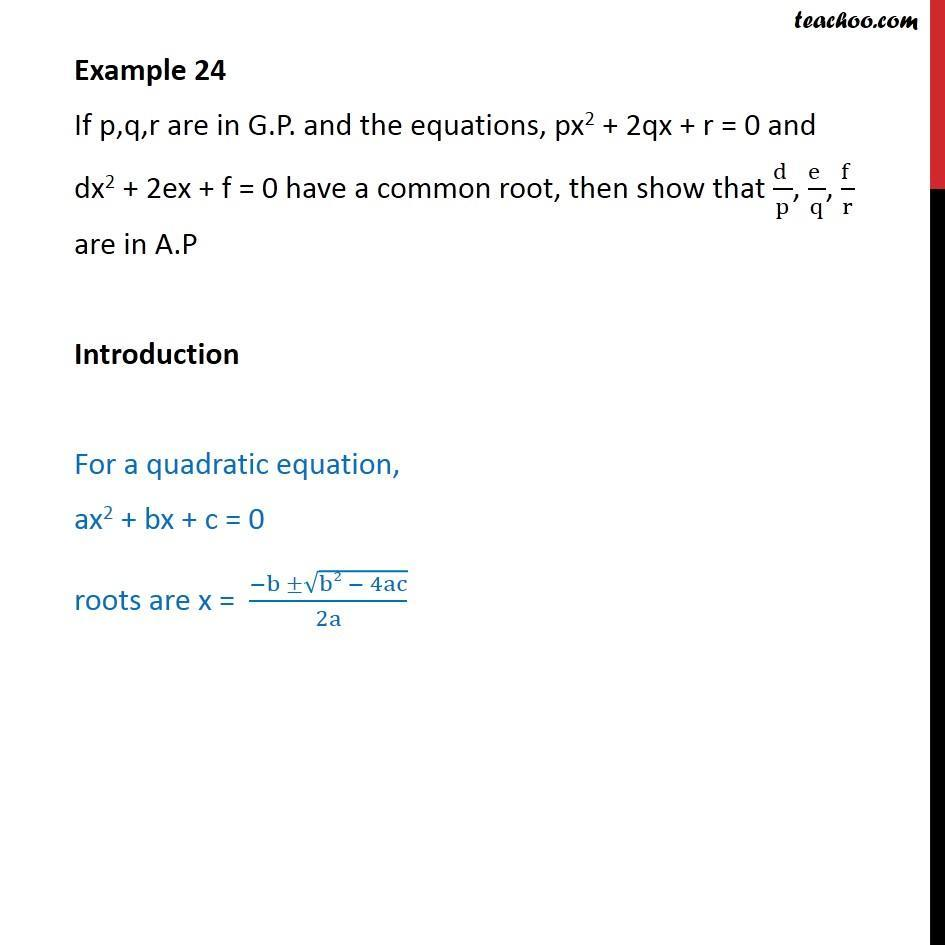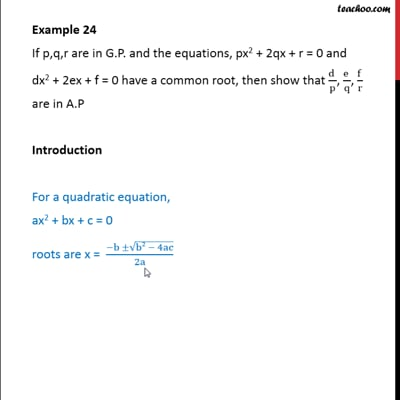This video is only available for Teachoo black users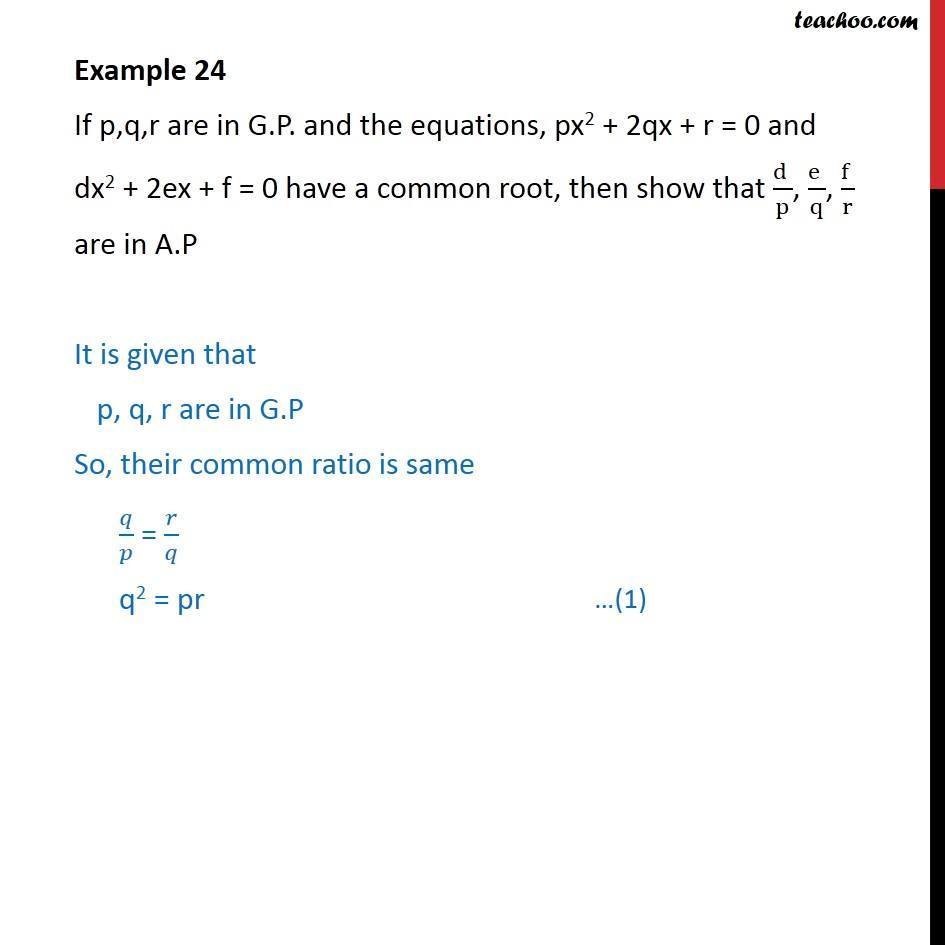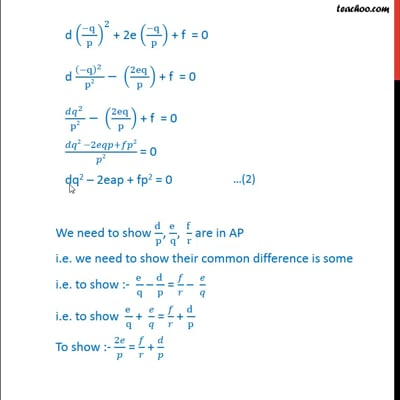This video is only available for Teachoo black users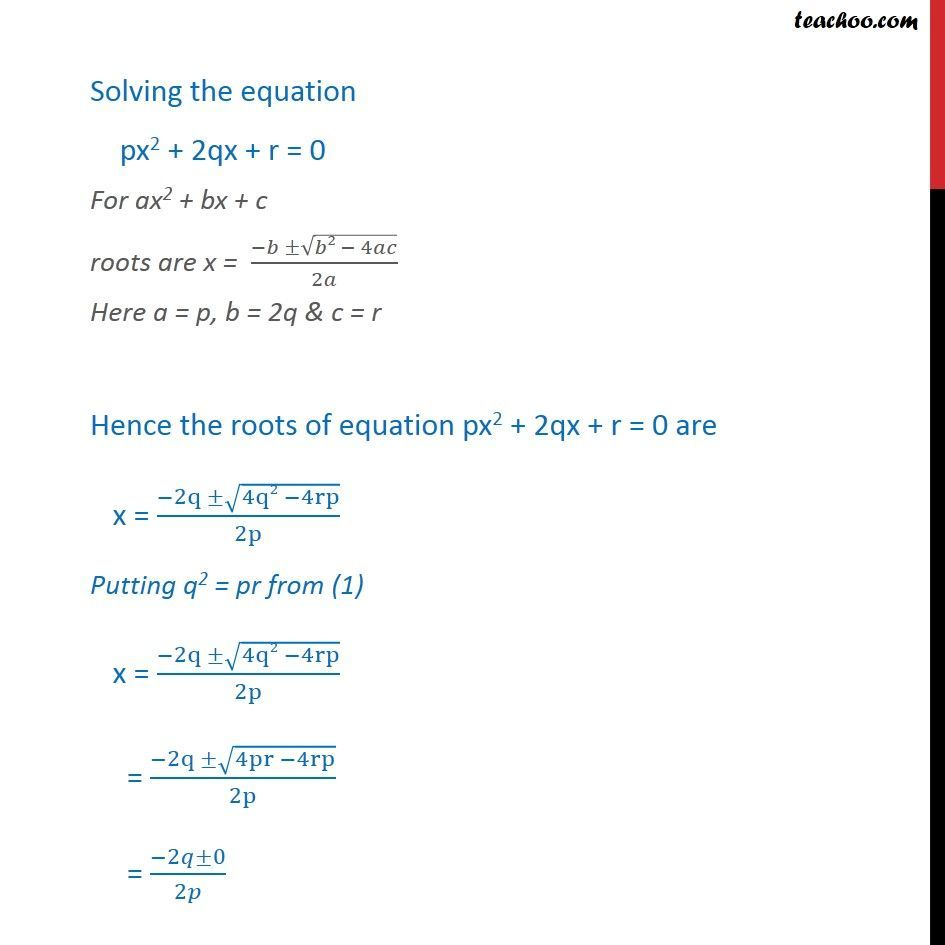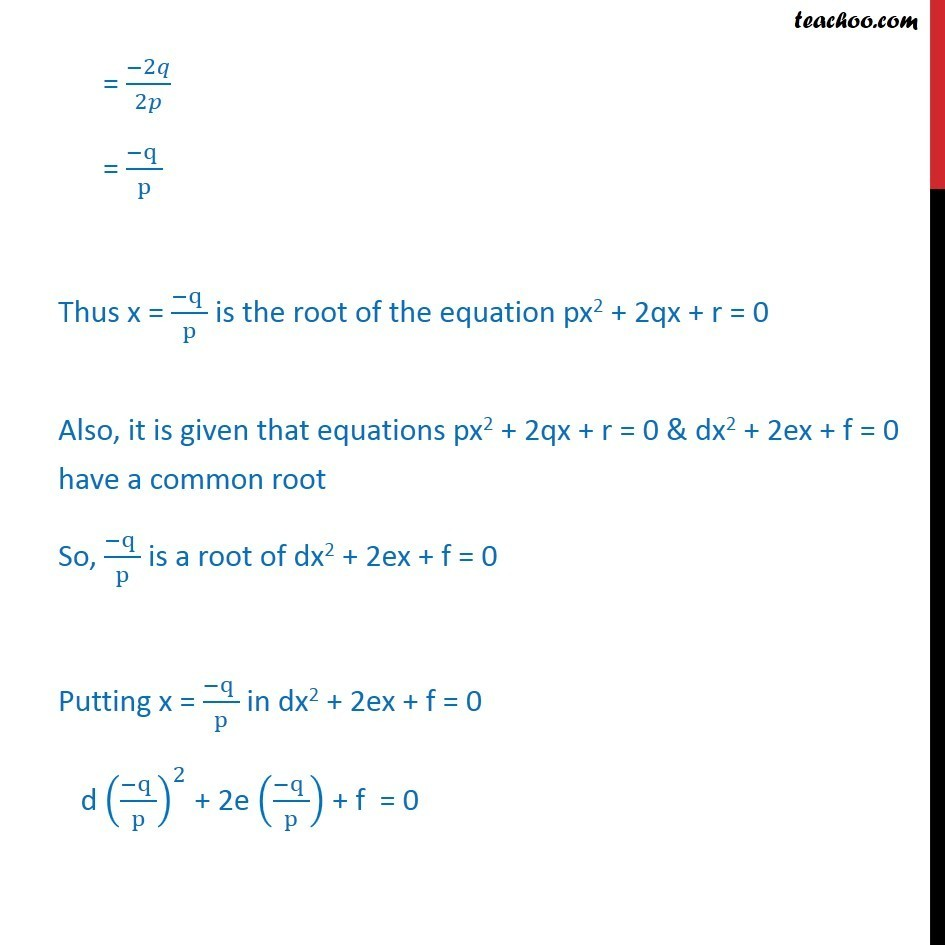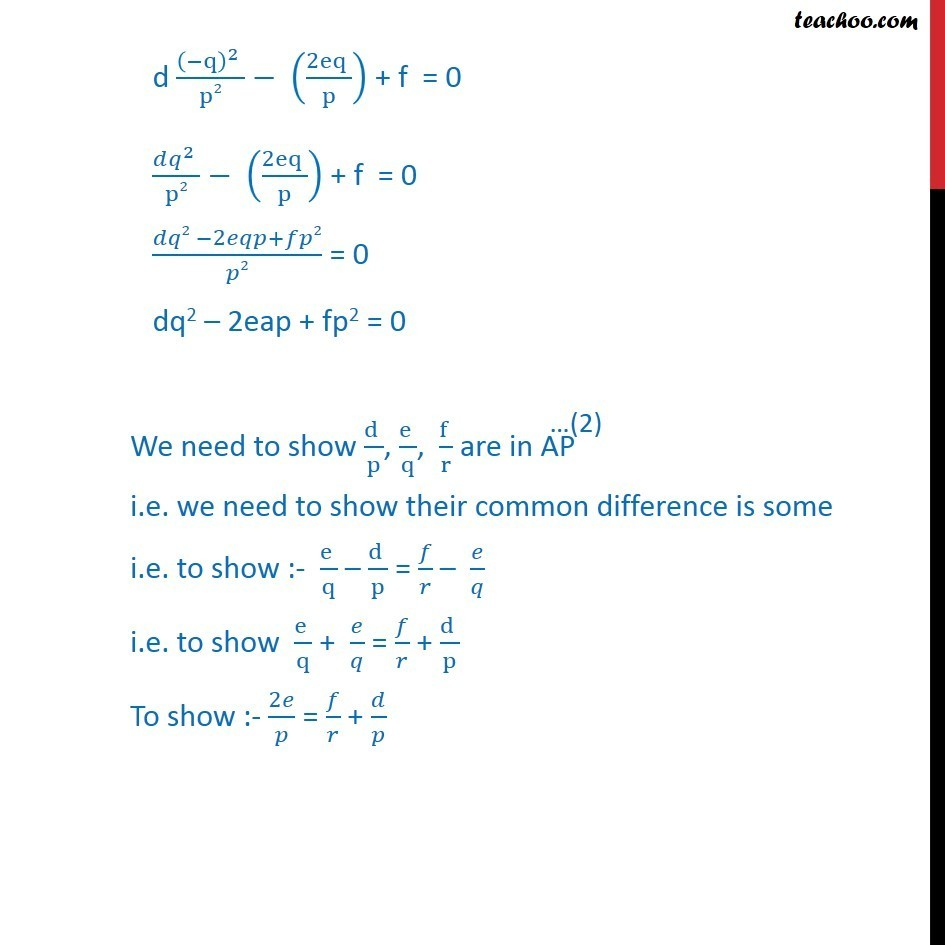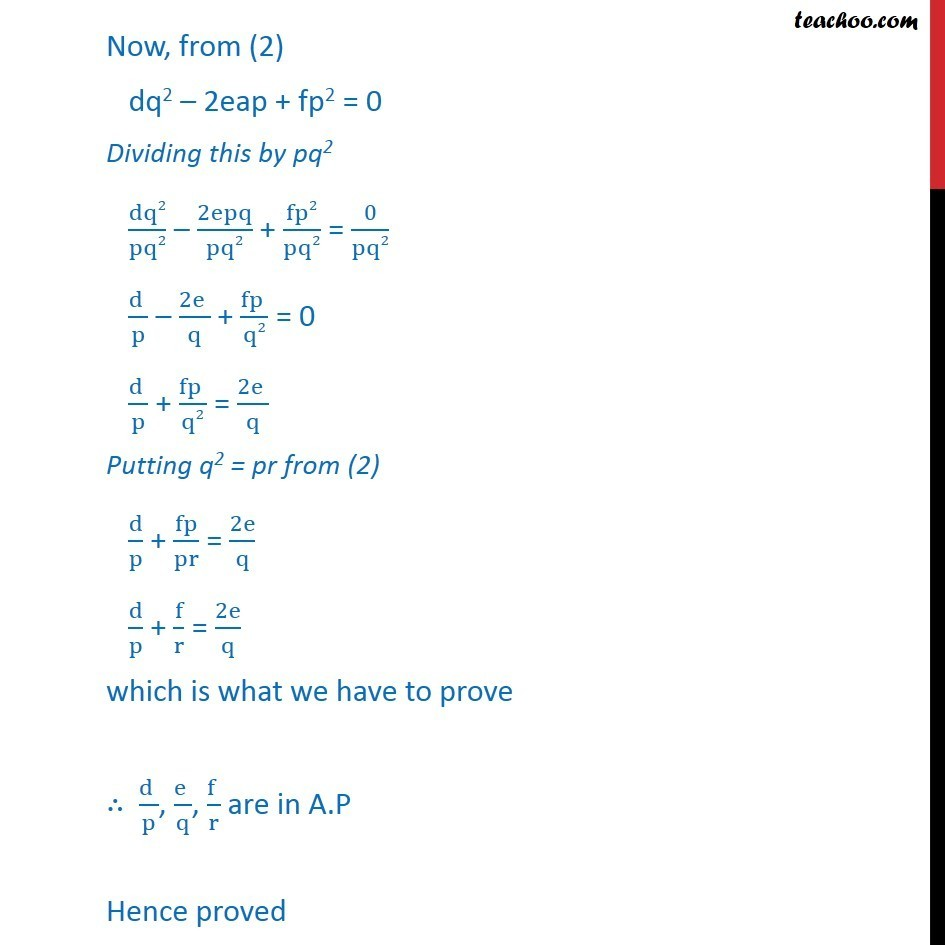### Transcript

Example 24 If p,q,r are in G.P. and the equations, px2 + 2qx + r = 0 and dx2 + 2ex + f = 0 have a common root, then show that (d )/p, (e )/q, (f )/r are in A.P It is given that p, q, r are in G.P So, their common ratio is same / = / q2 = pr Solving the equation px2 + 2qx + r = 0 For ax2 + bx + c roots are x = ( ( 2 4 ))/2 Here a = p, b = 2q & c = r Hence the roots of equation px2 + 2qx + r = 0 are x = ( 2q (4q2 4rp))/2p Putting q2 = pr from (1) x = ( 2q (4q2 4rp))/2p = ( 2q (4pr 4rp))/2p = ( 2 0)/2 = ( 2 )/2 = ( q )/p Thus x = ( q )/p is the root of the equation px2 + 2qx + r = 0 Also, it is given that equations px2 + 2qx + r = 0 & dx2 + 2ex + f = 0 have a common root So, ( q )/p is a root of dx2 + 2ex + f = 0 Putting x = ( q )/p in dx2 + 2ex + f = 0 d (( q )/p)^2 + 2e (( q )/p) + f = 0 d (( q)^2 )/p2 ((2eq )/p) + f = 0 ( ^2 )/p2 ((2eq )/p) + f = 0 ( 2 2 + 2)/ 2 = 0 dq2 2eap + fp2 = 0 We need to show (d )/p, (e )/q, (f )/r are in AP i.e. we need to show their common difference is some i.e. to show :- (e )/q (d )/p = / / i.e. to show (e )/q + / = / + (d )/p To show :- 2 / = / + / Now, from (2) dq2 2eap + fp2 = 0 Dividing this by pq2 dq2/pq2 2epq/pq2 + fp2/pq2 = 0/pq2 (d )/p (2e )/q + (fp )/q2 = 0 (d )/p + (fp )/q2 = (2e )/q Putting q2 = pr from (2) d/p + fp/pr = 2e/q d/p + f/r = 2e/q which is what we have to prove (d )/p, (e )/q, (f )/r are in A.P Hence proved